### Knowledge and Uncertainty

You run the mint for a small kingdom and the Queen has requested $4$ coins for her trip abroad (she is a modest ruler). Everything is in place when, on the eve of your delivery, thieves break into the mint.

In the morning, you find the Queen's coins along with a note:

These pretty coins shine in the light.
But so does a fake and just as bright.
Did I gaze at them then take my leave,
Or replace one with a forgery?

If you can't read old English, here's a translation: it's possible that one of the coins is a forgery.

To help to figure it out, you're given a scale and a reference coin that's guaranteed to be real.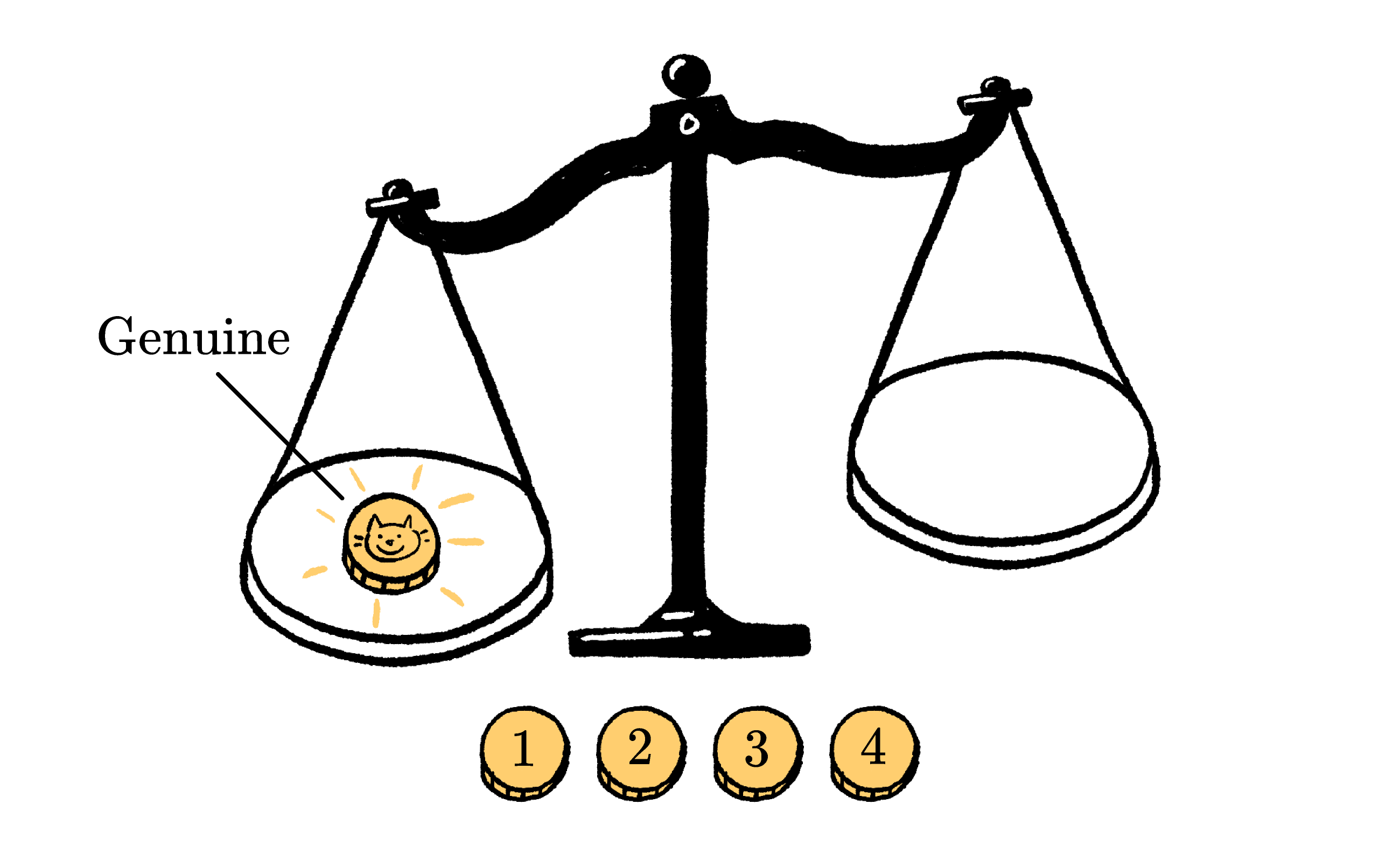How many times do you need to use the scale to find out the truth?

# Find the Counterfeit Coin

Remember — in this puzzle there are $4$ coins, and either one of them is counterfeit, or all of them are real.

If one of the coins is counterfeit, it can either be heavier or lighter than the others.

For example, one of the possibilities is "coin $3$ is the counterfeit and weighs less than a genuine coin." Another possibility is "all the coins are real."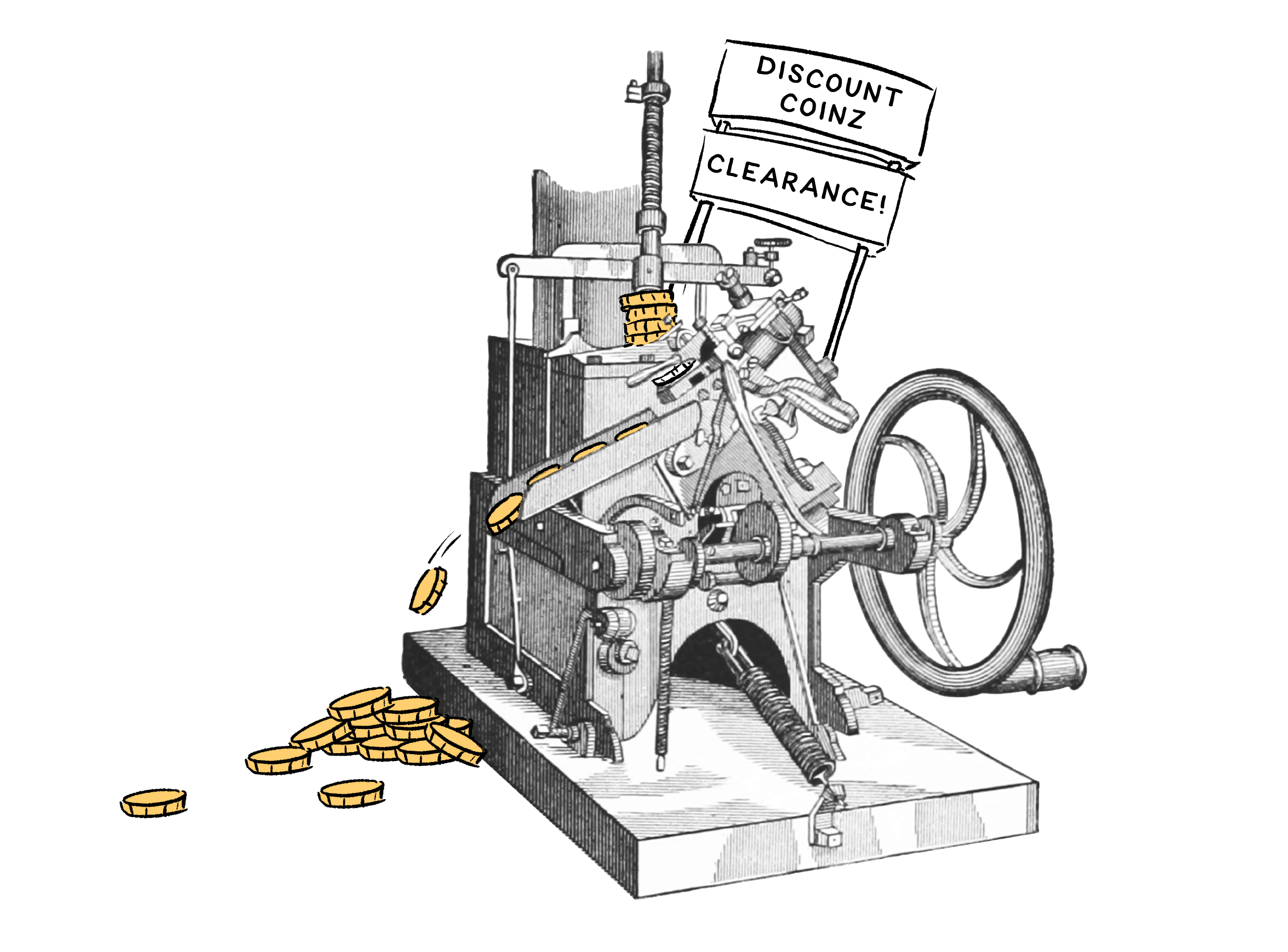How many possibilities are there?

# Find the Counterfeit Coin

There are nine possible answers that we need to narrow down.

We can give each of them a label so that, for example,

• "coin $3$ is the counterfeit and is lighter than a real coin" $\rightarrow \textcolor{#3D99F6}{\textbf{3}^-}$ and
• "all the coins are real" $\rightarrow \textcolor{#69047E}{\textbf{A}}.$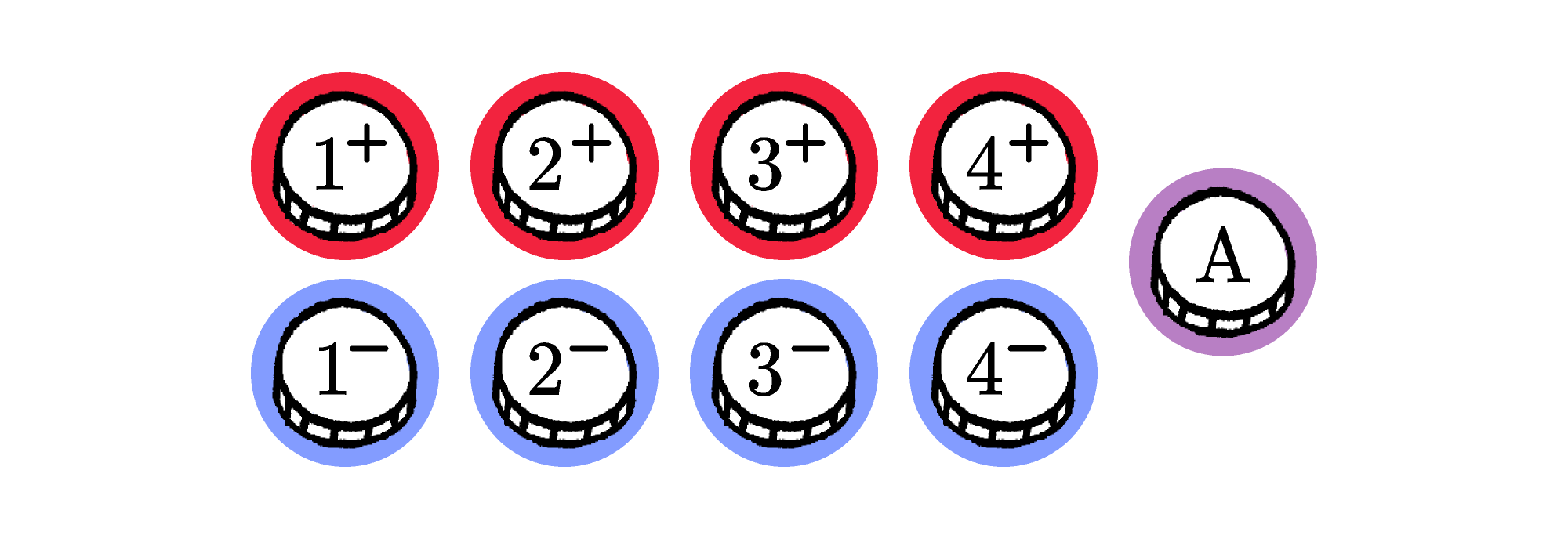If each of these outcomes is equally likely, what is the probability of $\textcolor{#69047E}{\textbf{A}}?$

# Find the Counterfeit Coin

It might be tempting to start our weighing by putting half the coins on one side and the other half on the other: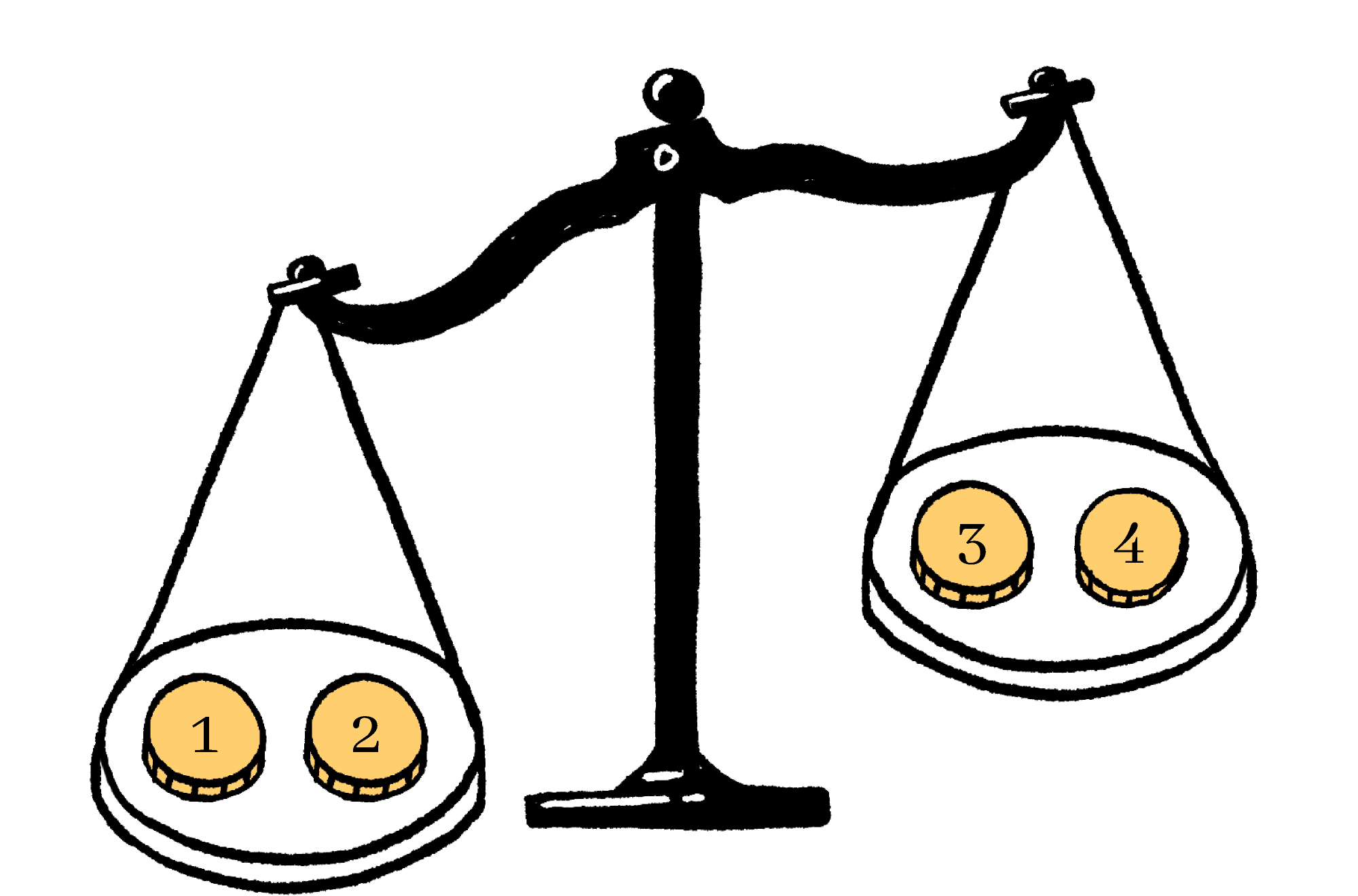Suppose we do this and find that the left side is heavier. Which hypotheses might still be true?

Remember that these were the nine hypotheses originally:# Find the Counterfeit Coin

This picture shows us which hypotheses would remain after each of the possible outcomes: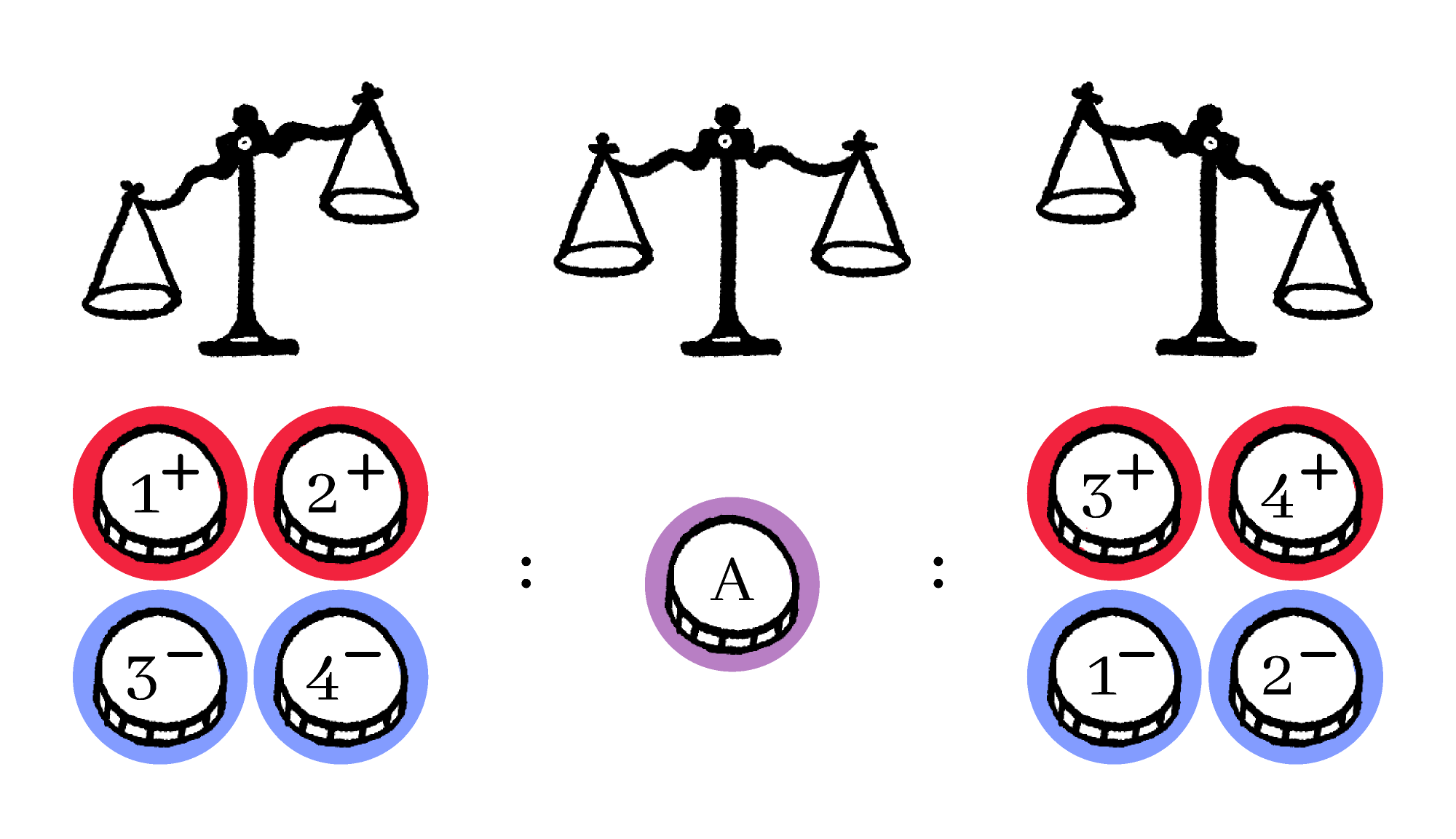It seems that there aren't an equal number of hypotheses left standing in each case.

# Find the Counterfeit Coin

We can judge it by quantifying how many hypotheses are still possible, on average, after we get the answer. We'd like to make that number as low as possible because we need to try to eliminate possibilities using as few questions as possible.Find the average number of hypotheses left, given that

• $\frac19$ of the time you're left with $1$ hypothesis and
• $\frac89$ of the time you're left with $4$ hypotheses.

# Find the Counterfeit Coin

Suppose you're about to put a group of coins on the left side of a scale, and another group on the right side. One possibility is that the left side will be heavier than the right.

How many possible outcomes are there for this weighing in total?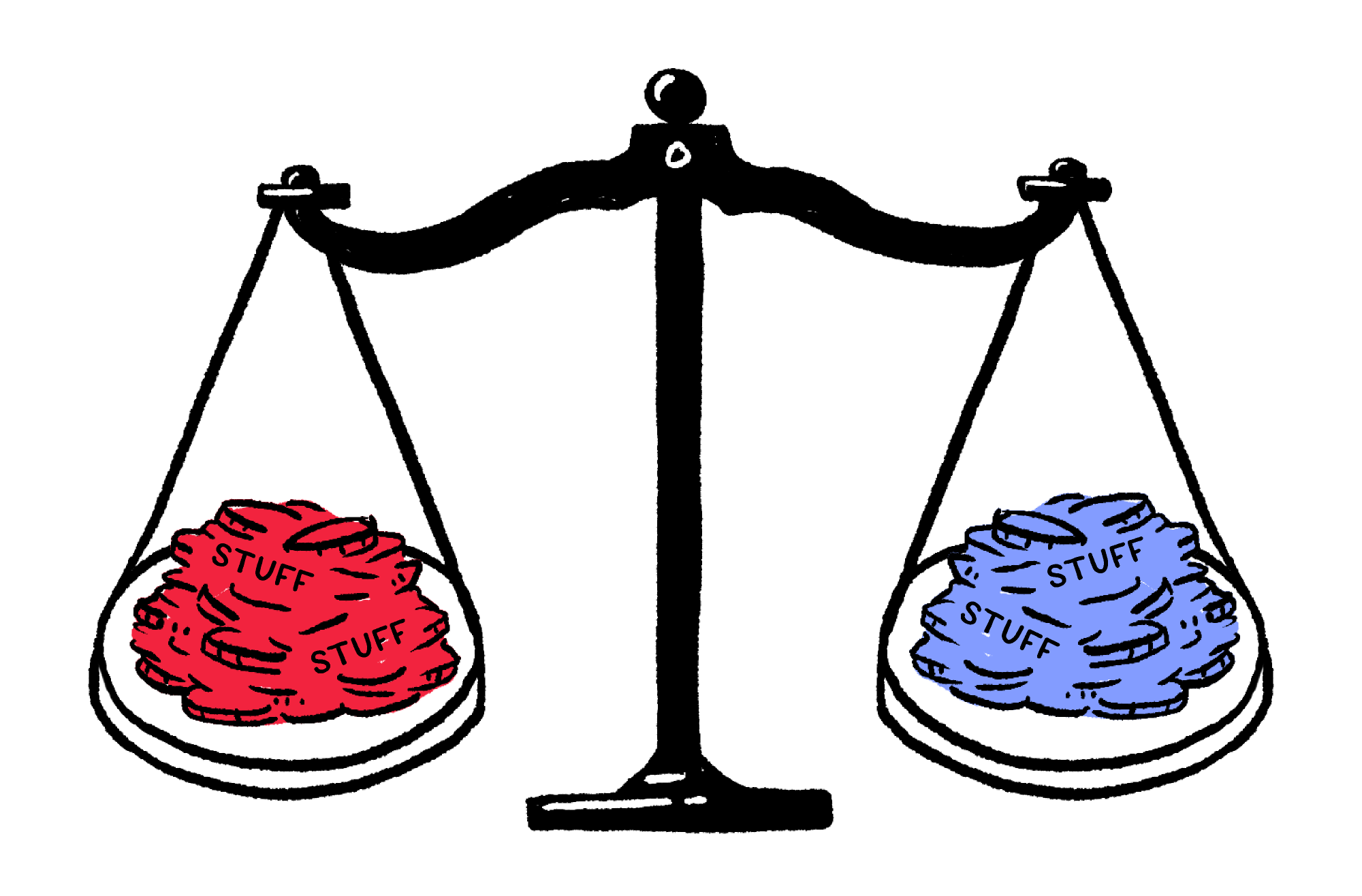# Find the Counterfeit Coin

What makes for an informative weighing in general?

When you weigh two things against each other, you're really asking, "How do the weights of these two objects compare?" There are three possible answers:

1. the left is heavier than the right,
2. the right is heavier than the left, or
3. both sides weigh the same.

This was the insight from our last quiz:

The most informative questions are the ones whose answer we are the most uncertain about.

The first weighing we considered did not obey this principle, since there was one thing we could be fairly confident about — both sides would not weigh the same. That's why that weighing was not maximally informative.

We'd have the most uncertainty if all three possible answers were equally likely, instead.

How many hypotheses would be left if we asked such a maximally informative question?# Find the Counterfeit Coin

Our original weighing left behind more hypotheses on average than a maximally informative question would have $($i.e. about $3.67$ rather than just $3).$

This is why we're looking for a weighing that splits the hypotheses into three groups of equal size.Which of these weighings would do that?

To clarify, as in pane $1$ of this quiz, a coin with a cat face is a reference coin.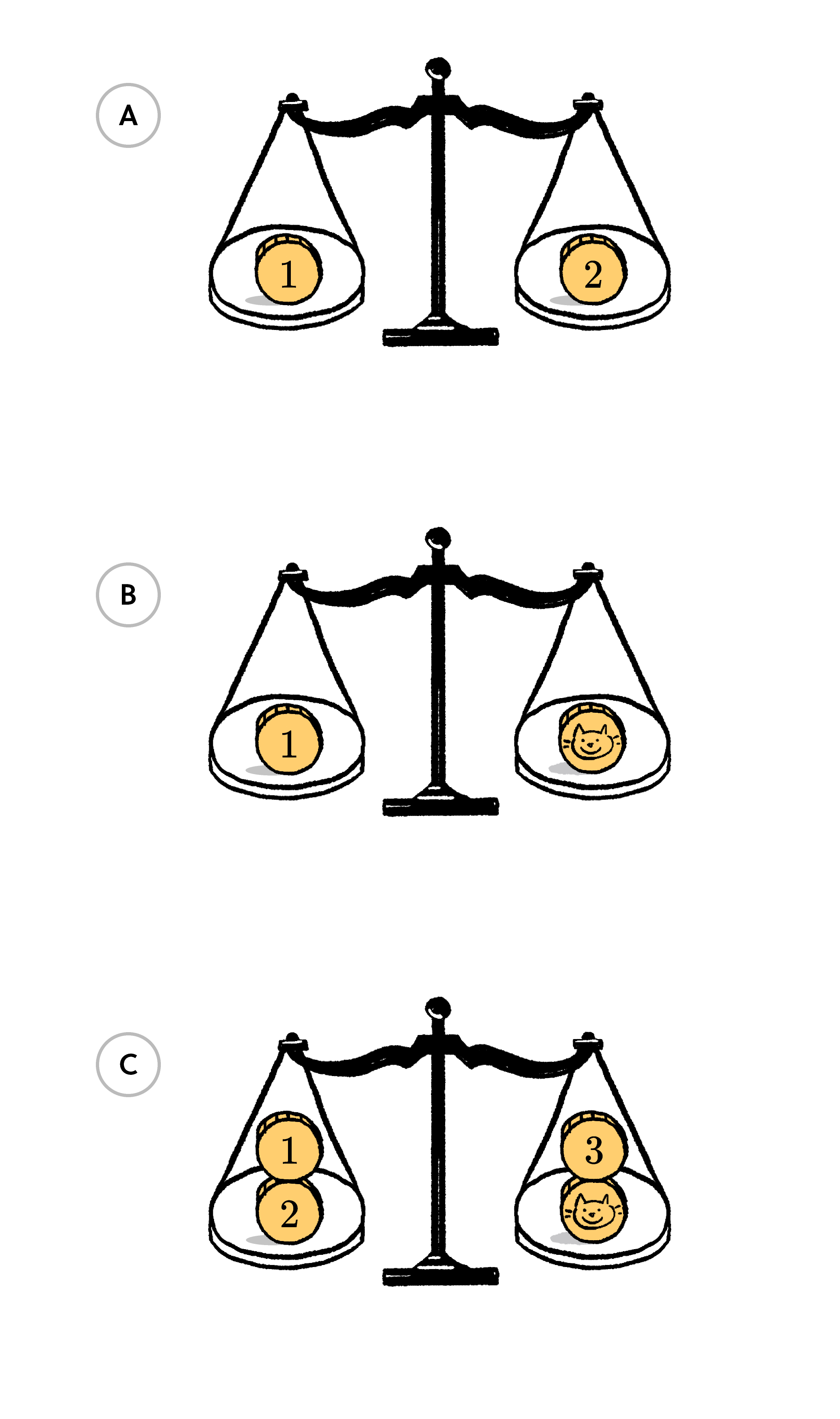# Find the Counterfeit Coin

Let's see why this weighing worked: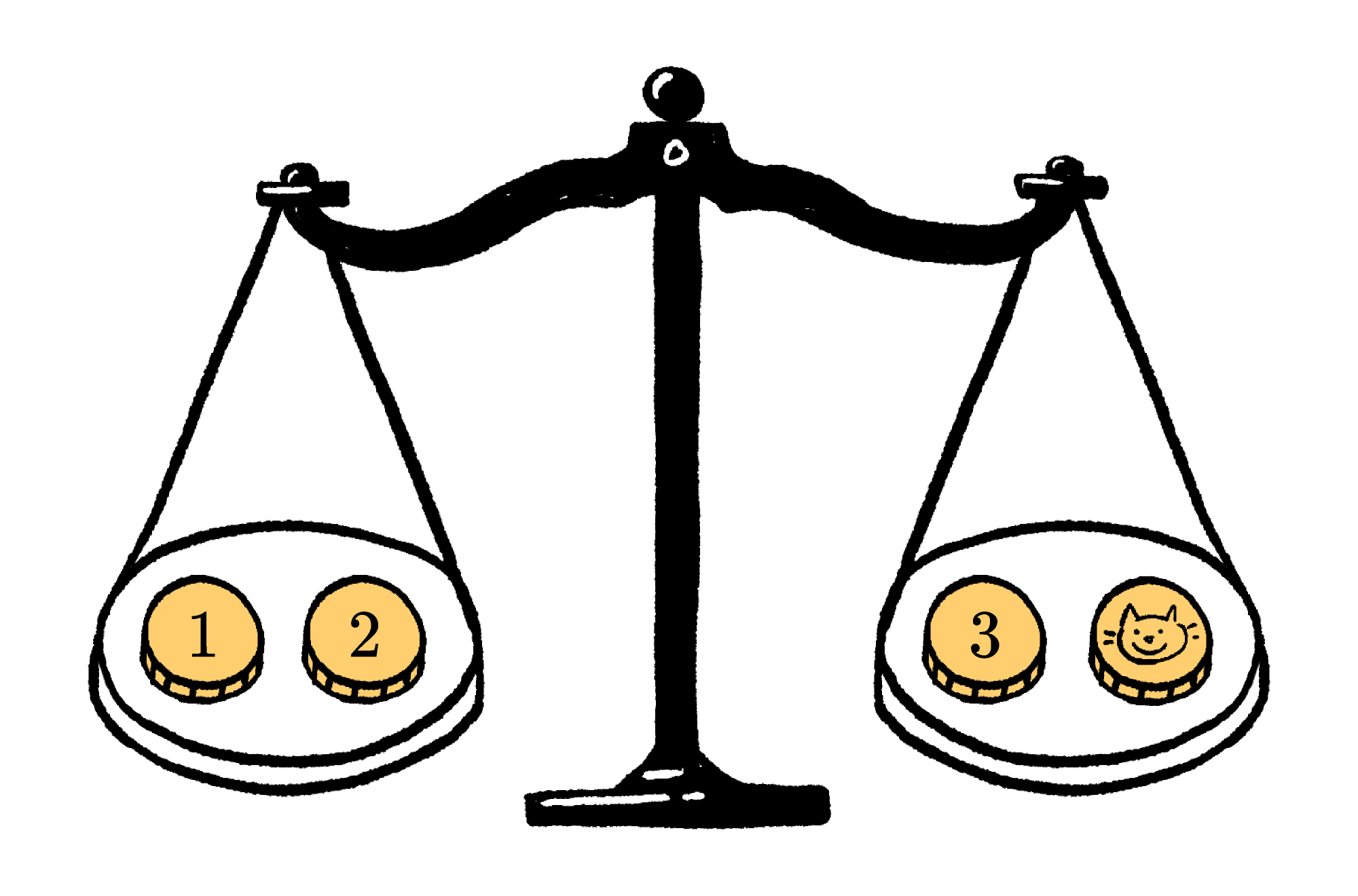If we leave off coin $4,$ there are now more ways for the two sides to balance — either there are no counterfeit coins, or coin $4$ is the counterfeit and it's heavy or light.

If the left side is heavier, then that means that either coin $1$ or $2$ is counterfeit and heavier, or else coin $3$ is counterfeit and it's lighter. This way, the scale partitions the hypotheses into three equal-sized groups: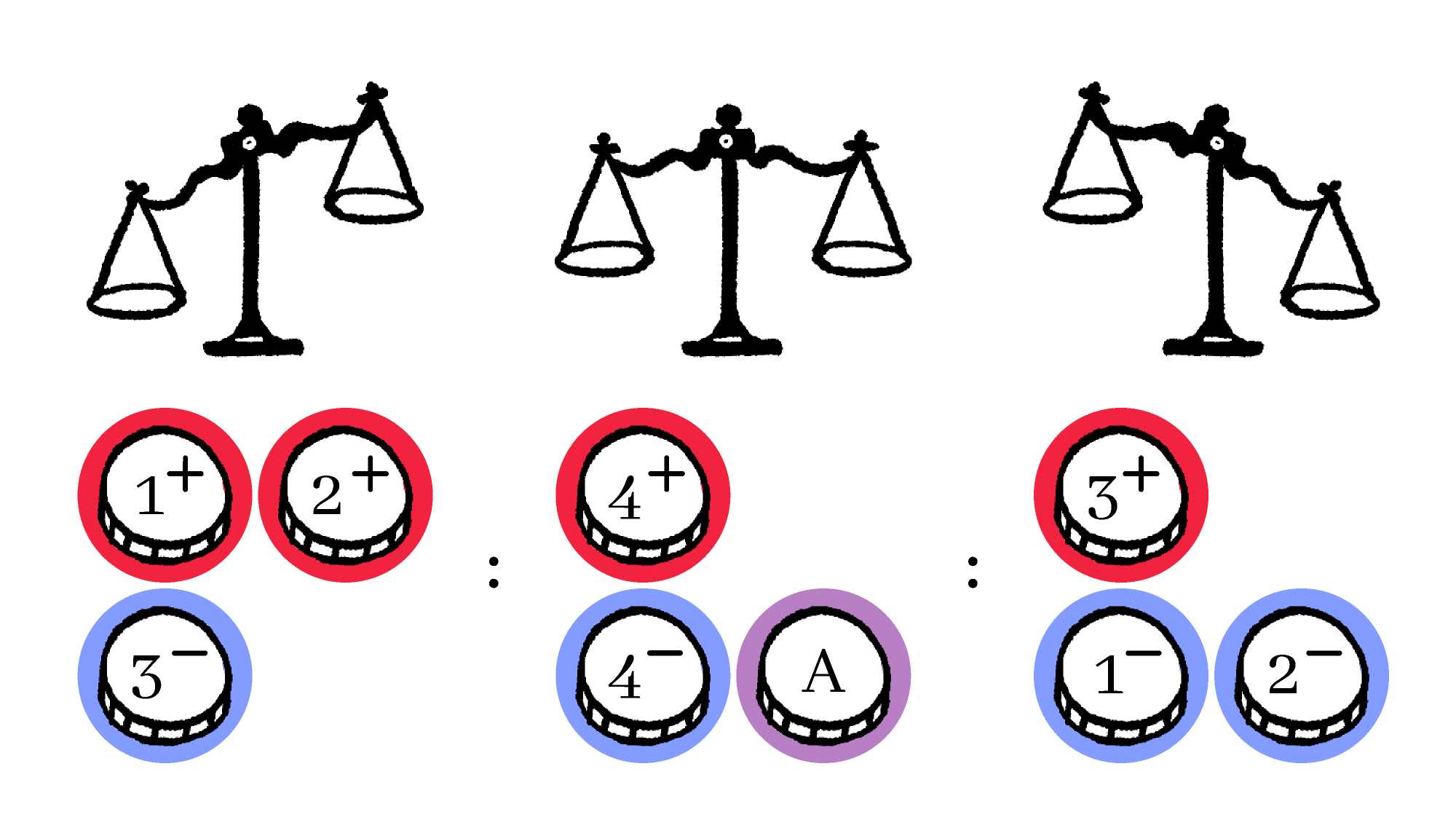# Find the Counterfeit Coin

Suppose we make this measurement and find that the left side is heavier, leaving these three possibilities: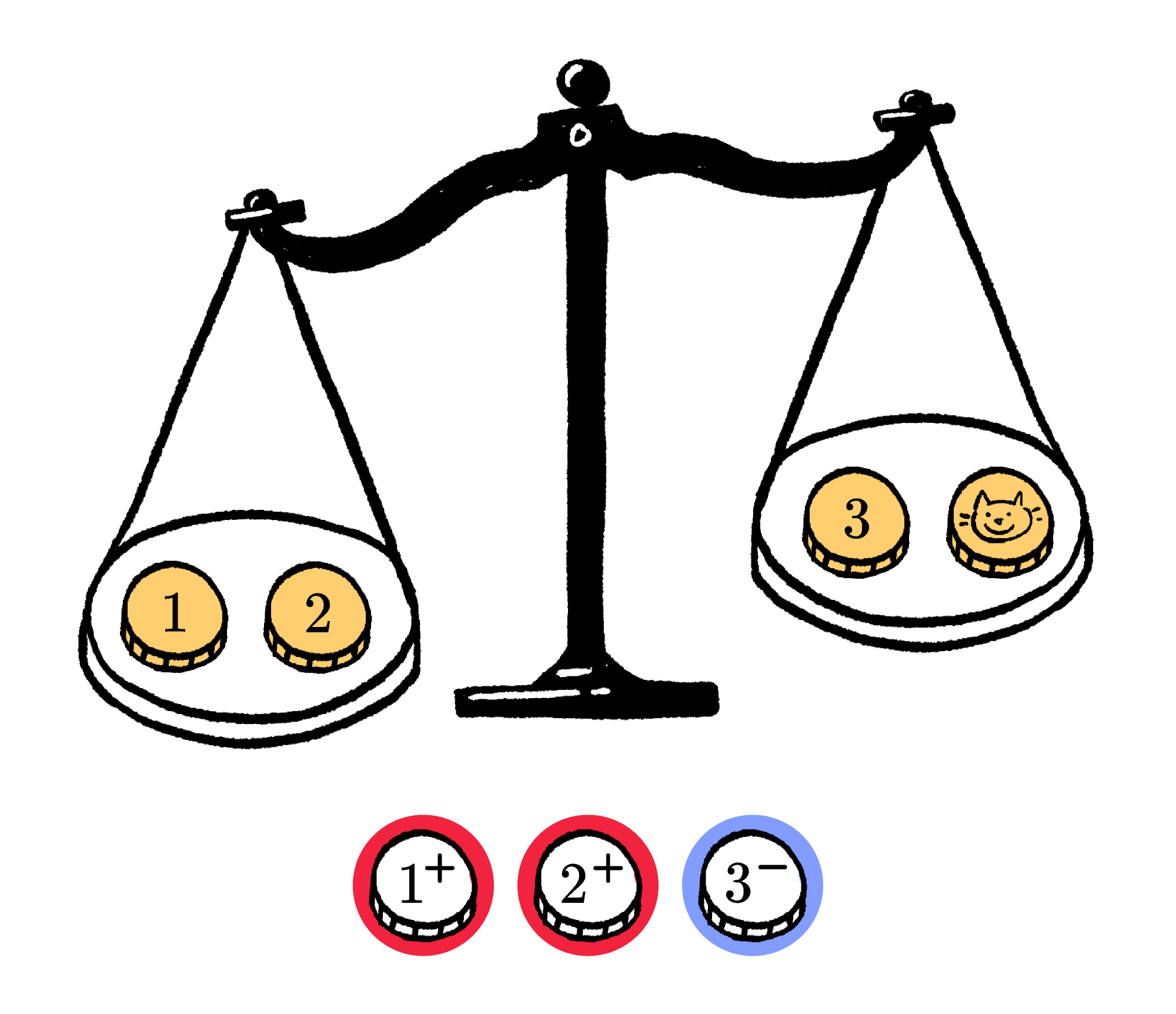Which of these should be our second weighing?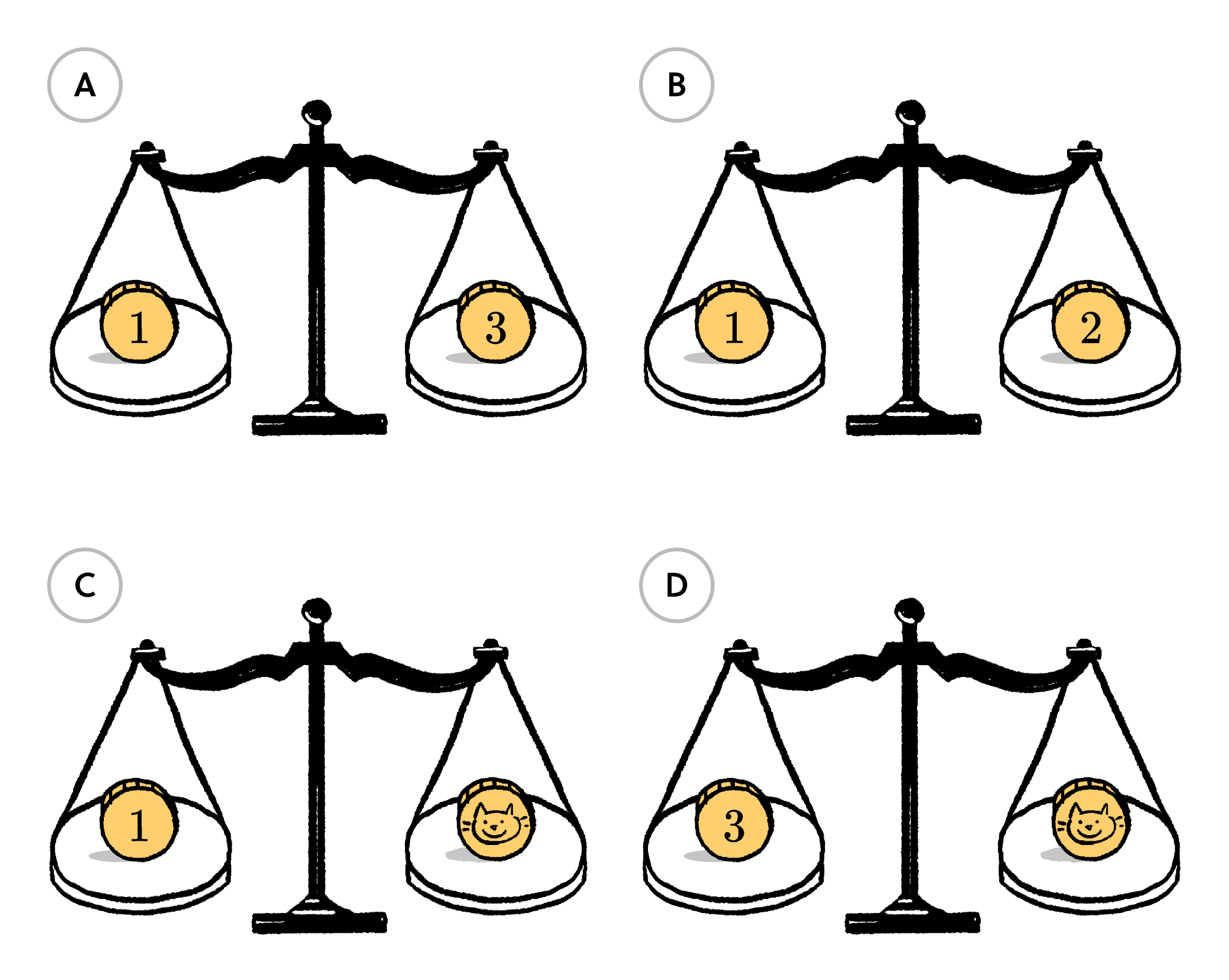# Find the Counterfeit Coin

When we did this weighing, both sides balanced!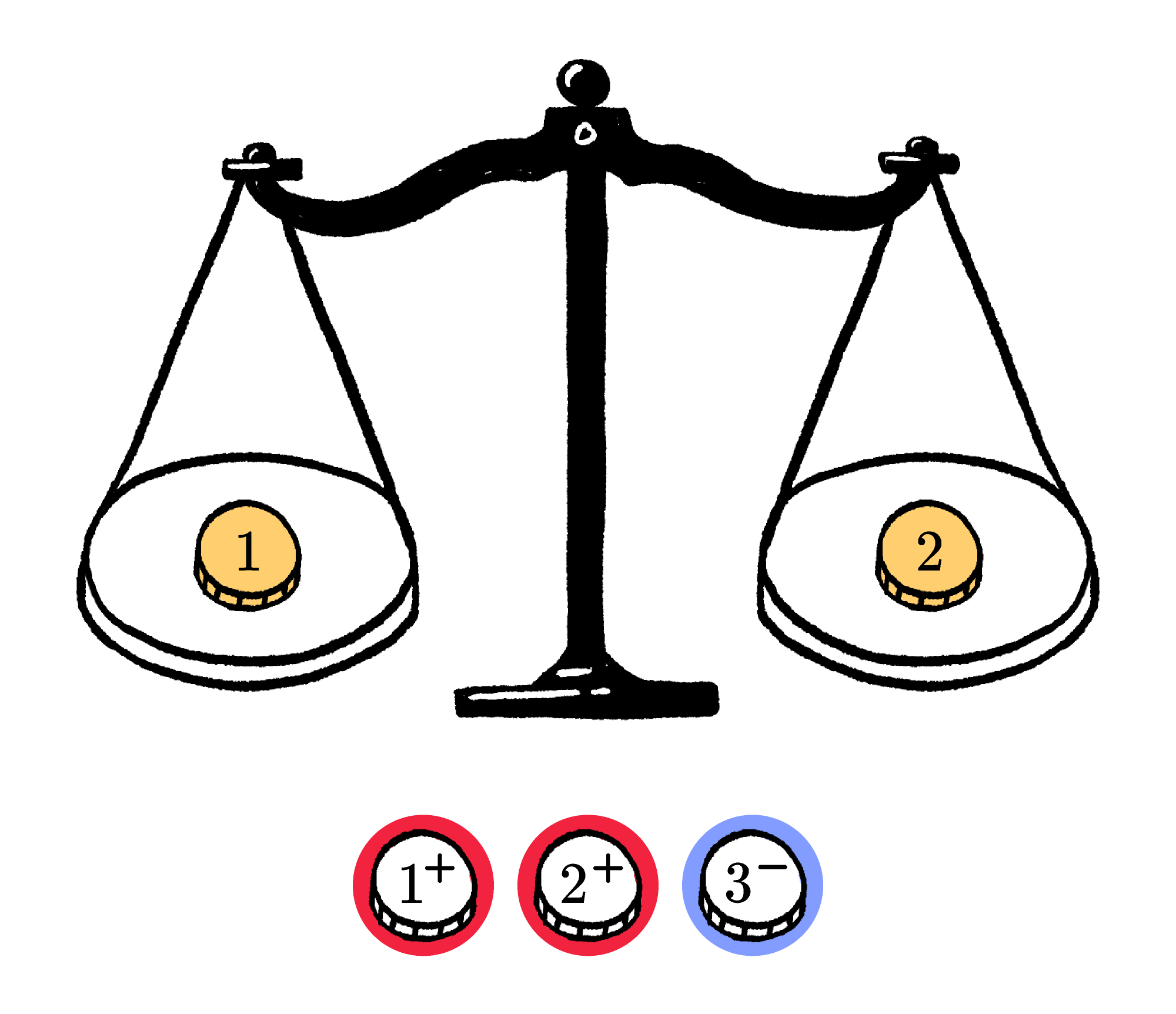Which coin is the counterfeit? And is it heavy or light?

# Find the Counterfeit Coin

Well done, you found the counterfeit coin!The solution turned out not to be arbitrary at all. All we had to use was our insight:

The most informative questions are the ones whose answer we are most uncertain about.

# Find the Counterfeit Coin

In fact, this insight is enough to answer a much harder version of the puzzle.

Suppose that you have $13$ coins that you must investigate and, as before, one real coin and a scale to help you. Can you figure out the least number of weighings to find the counterfeit coin (if there is one) and know whether it is heavy or light?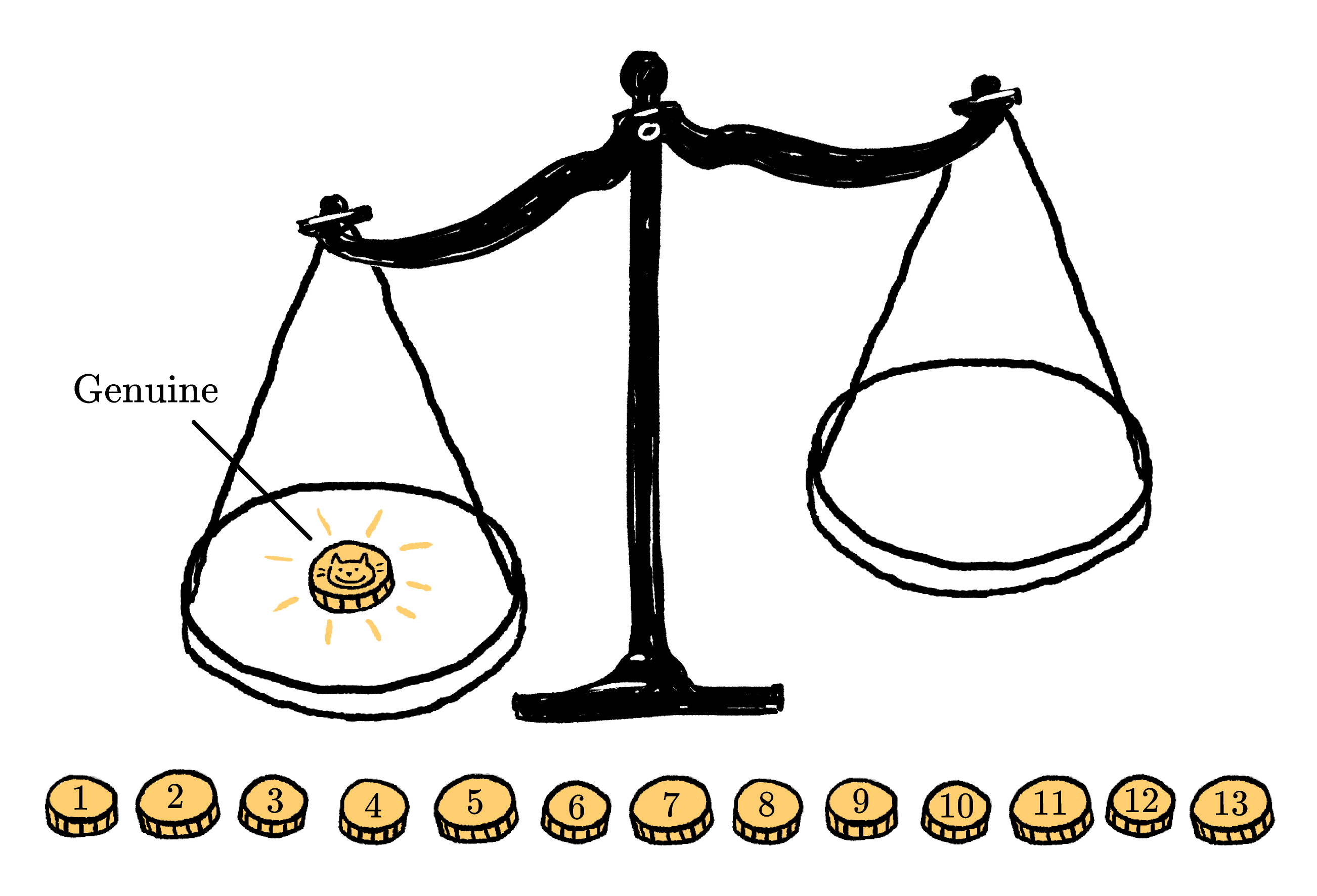You can try to answer this question yourself with what we've learned, or you can wait until we revisit this problem in Chapter 2.

# Find the Counterfeit Coin

×